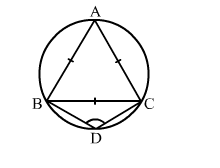# In the given figure, equilateral ∆ABC is inscribed in a circle and ABCD is a quadrilateral,

Question:

In the given figure, equilateral ABC is inscribed in a circle and ABCD is a quadrilateral, as shown. Then, ∠BDC = ?
(a) 90°

(b) 60°
(c) 120°
(d) 150°Solution:

(c) 120°
Since ΔABC is an equilateral triangle, each of its angle is 60°.
∴ BAC = 60°
In a cyclic quadrilateral ABCD, we have:
BAC + BDC = 180°
⇒ 60° + BDC = 180°
⇒ BDC = (180° - 60°) = 120°